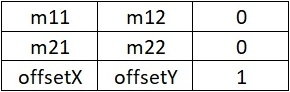﻿ L_MATRIX | LEADTOOLS Primitive Basic Data Types | Raster Imaging C API Help
In This Topic ▼

# L_MATRIX

````struct L_MATRIX `
`{ `
`   L_DOUBLE m11; `
`   L_DOUBLE m12; `
`   L_DOUBLE m21; `
`   L_DOUBLE m22; `
`   L_DOUBLE offsetX; `
`   L_DOUBLE offsetY; `
`   L_INT type; `
`   L_INT padding; `
`}; `
`typedef struct L_MATRIX L_MATRIX; ````

Represents a 3x3 affine transformation matrix used for transformations in 2-D space.

## Members

### m11

The value of the first row and first column.

### m12

The value of the first row and second column.

### m21

The value of the second row and first column.

### m22

The value of the second row and second column.

### offsetX

The x translation value (the dx value, or the element in the third row and first column).

### offsetY

The y translation value (the dy value, or the element in the third row and second column).

### type

Internal use only.

Internal use only.

3x3 matrix is used for transformations in a 2-D x-y plane. Affine transformation matrices can be multiplied to form any number of linear transformations, such as rotation and skew (shear), followed by translation. An affine transformation matrix has its final column equal to (0, 0, 1), so only the members in the first two columns need to be specified. Note that vectors are expressed as row-vectors, not column vectors.

The L_MATRIX is stored using row-major order and has the following structure:members in the last row, offsetX and offsetY, represent translation values.

In functions and structure memebers, the transformation matrix is usually specified as a vector with only six members, as follows:

(m11, m12, m21, m22, offsetX, offsetY)

The structure is used by:

Help Version 20.0.2019.12.5